Physics - Law of Conservation of Energy - With Example

Law of Conservation of Energy

Statement :

Energy can neither be created nor destroyed but one form of energy can be transformed in to another form of energy without any loss or gain of energy.

The total energy that exists in the universe in all form remain constant.

Example :

In a battery chemical energy is converte to electrical energy.

In a dynamo, mechanical energy is converted in to electrical energy.

Verification of law of conservation of energy in case of a freely falling body:

Consider a body of mass 'm' released from Point 'A' above the surface of the earth at height 'h'.

As the body falls freely under gravity , its P.E decreases .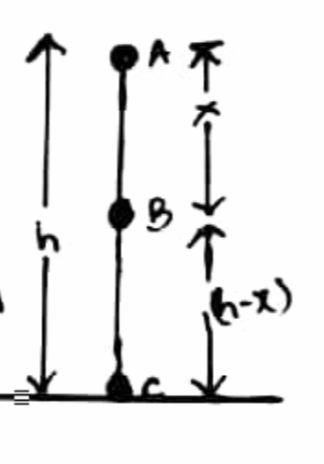At A :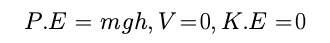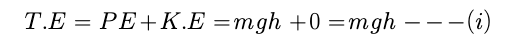At B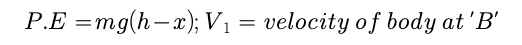we know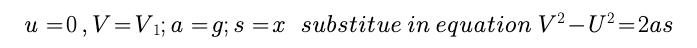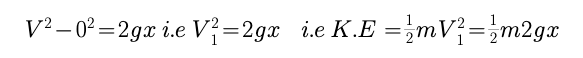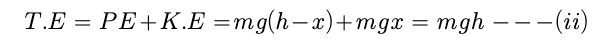At point 'c'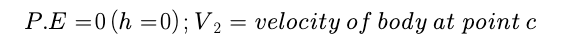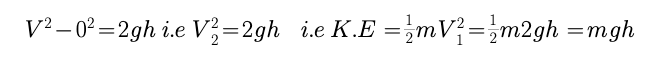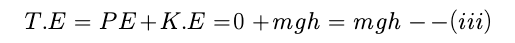T.E is same at point A, B, C = mgh

Post By : Rahul Kumar 30 May, 2020 335 views Physics